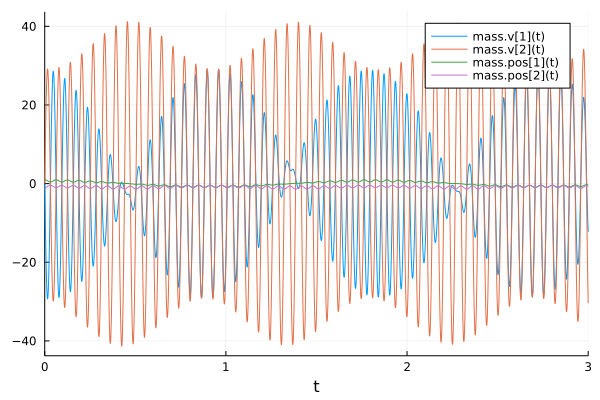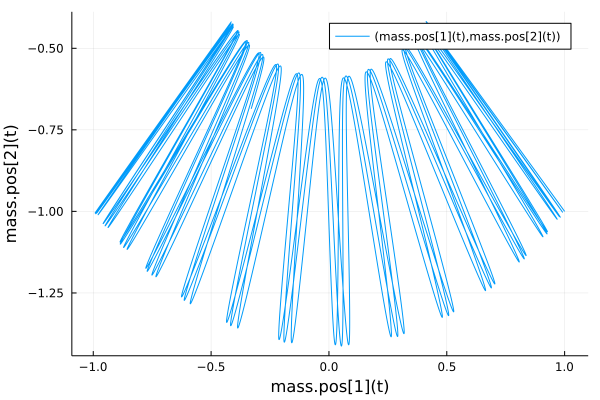# Component-Based Modeling a Spring-Mass System

In this tutorial we will build a simple component-based model of a spring-mass system. A spring-mass system consists of one or more masses connected by springs. Hooke's law gives the force exerted by a spring when it is extended or compressed by a given distance. This specifies a differential-equation system where the acceleration of the masses is specified using the forces acting on them.

## Copy-Paste Example

using ModelingToolkit, Plots, DifferentialEquations, LinearAlgebra

@variables t
D = Differential(t)

function Mass(; name, m = 1.0, xy = [0., 0.], u = [0., 0.])
ps = @parameters m=m
sts = @variables pos[1:2](t)=xy v[1:2](t)=u
eqs = collect(D.(pos) .~ v)
ODESystem(eqs, t, [pos..., v...], ps; name)
end

function Spring(; name, k = 1e4, l = 1.)
ps = @parameters k=k l=l
@variables x(t), dir[1:2](t)
ODESystem(Equation[], t, [x, dir...], ps; name)
end

function connect_spring(spring, a, b)
[
spring.x ~ norm(collect(a .- b))
collect(spring.dir .~ collect(a .- b))
]
end

spring_force(spring) = -spring.k .* collect(spring.dir) .* (spring.x - spring.l)  ./ spring.x

m = 1.0
xy = [1., -1.]
k = 1e4
l = 1.
center = [0., 0.]
g = [0., -9.81]
@named mass = Mass(m=m, xy=xy)
@named spring = Spring(k=k, l=l)

eqs = [
connect_spring(spring, mass.pos, center)
collect(D.(mass.v) .~ spring_force(spring) / mass.m .+ g)
]

@named _model = ODESystem(eqs, t)
@named model = compose(_model, mass, spring)
sys = structural_simplify(model)

prob = ODEProblem(sys, [], (0., 3.))
sol = solve(prob, Rosenbrock23())
plot(sol)## Explanation

### Building the components

For each component we use a Julia function that returns an ODESystem. At the top, we define the fundamental properties of a Mass: it has a mass m, a position pos and a velocity v. We also define that the velocity is the rate of change of position with respect to time.

function Mass(; name, m = 1.0, xy = [0., 0.], u = [0., 0.])
ps = @parameters m=m
sts = @variables pos[1:2](t)=xy v[1:2](t)=u
eqs = collect(D.(pos) .~ v)
ODESystem(eqs, t, [pos..., v...], ps; name)
end

Note that this is an incompletely specified ODESystem. It cannot be simulated on its own since the equations for the velocity v[1:2](t) are unknown. Notice the addition of a name keyword. This allows us to generate different masses with different names. A Mass can now be constructed as:

Mass(name = :mass1)

Or using the @named helper macro

@named mass1 = Mass()

Next we build the spring component. It is characterised by the spring constant k and the length l of the spring when no force is applied to it. The state of a spring is defined by its current length and direction.

function Spring(; name, k = 1e4, l = 1.)
ps = @parameters k=k l=l
@variables x(t), dir[1:2](t)
ODESystem(Equation[], t, [x, dir...], ps; name)
end

We now define functions that help construct the equations for a mass-spring system. First, the connect_spring function connects a spring between two positions a and b. Note that a and b can be the pos of a Mass, or just a fixed position such as [0., 0.]. In that sense, the length of the spring x is given by the length of the vector dir joining a and b.

function connect_spring(spring, a, b)
[
spring.x ~ norm(collect(a .- b))
collect(spring.dir .~ collect(a .- b))
]
end

Lastly, we define the spring_force function that takes a spring and returns the force exerted by this spring.

spring_force(spring) = -spring.k .* collect(spring.dir) .* (spring.x - spring.l)  ./ spring.x

To create our system, we will first create the components: a mass and a spring. This is done as follows:

m = 1.0
xy = [1., -1.]
k = 1e4
l = 1.
center = [0., 0.]
g = [0., -9.81]
@named mass = Mass(m=m, xy=xy)
@named spring = Spring(k=k, l=l)

We can now create the equations describing this system, by connecting spring to mass and a fixed point.

eqs = [
connect_spring(spring, mass.pos, center)
collect(D.(mass.v) .~ spring_force(spring) / mass.m .+ g)
]

Finally, we can build the model using these equations and components.

@named _model = ODESystem(eqs, t)
@named model = compose(_model, mass, spring)

We can take a look at the equations in the model using the equations function.

equations(model)

7-element Vector{Equation}:
Differential(t)(mass₊v(t)) ~ -spring₊k*spring₊dir(t)*(mass₊m^-1)*(spring₊x(t) - spring₊l)*(spring₊x(t)^-1)
Differential(t)(mass₊v(t)) ~ -9.81 - (spring₊k*spring₊dir(t)*(mass₊m^-1)*(spring₊x(t) - spring₊l)*(spring₊x(t)^-1))
spring₊x(t) ~ sqrt(abs2(mass₊pos(t)) + abs2(mass₊pos(t)))
spring₊dir(t) ~ mass₊pos(t)
spring₊dir(t) ~ mass₊pos(t)
Differential(t)(mass₊pos(t)) ~ mass₊v(t)
Differential(t)(mass₊pos(t)) ~ mass₊v(t)

The states of this model are:

states(model)

7-element Vector{Term{Real, Base.ImmutableDict{DataType, Any}}}:
mass₊v(t)
mass₊v(t)
spring₊x(t)
mass₊pos(t)
mass₊pos(t)
spring₊dir(t)
spring₊dir(t)

And the parameters of this model are:

parameters(model)

6-element Vector{Sym{Real, Base.ImmutableDict{DataType, Any}}}:
spring₊k
mass₊m
spring₊l
mass₊m
spring₊k
spring₊l

### Simplifying and solving this system

This system can be solved directly as a DAE using one of the DAE solvers from DifferentialEquations.jl. However, we can symbolically simplify the system first beforehand. Running structural_simplify eliminates unnecessary variables from the model to give the leanest numerical representation of the system.

sys = structural_simplify(model)
equations(sys)

4-element Vector{Equation}:
Differential(t)(mass₊v(t)) ~ -spring₊k*mass₊pos(t)*(mass₊m^-1)*(sqrt(abs2(mass₊pos(t)) + abs2(mass₊pos(t))) - spring₊l)*(sqrt(abs2(mass₊pos(t)) + abs2(mass₊pos(t)))^-1)
Differential(t)(mass₊v(t)) ~ -9.81 - (spring₊k*mass₊pos(t)*(mass₊m^-1)*(sqrt(abs2(mass₊pos(t)) + abs2(mass₊pos(t))) - spring₊l)*(sqrt(abs2(mass₊pos(t)) + abs2(mass₊pos(t)))^-1))
Differential(t)(mass₊pos(t)) ~ mass₊v(t)
Differential(t)(mass₊pos(t)) ~ mass₊v(t)

We are left with only 4 equations involving 4 state variables (mass.pos, mass.pos, mass.v, mass.v). We can solve the system by converting it to an ODEProblem in mass matrix form and solving with an ODEProblem mass matrix solver. This is done as follows:

prob = ODEProblem(sys, [], (0., 3.))
sol = solve(prob, Rosenbrock23())
plot(sol)

What if we want the timeseries of a different variable? That information is not lost! Instead, structural_simplify simply changes state variables into observed variables.

observed(sys)

3-element Vector{Equation}:
spring₊dir(t) ~ mass₊pos(t)
spring₊dir(t) ~ mass₊pos(t)
spring₊x(t) ~ sqrt(abs2(mass₊pos(t)) + abs2(mass₊pos(t)))

These are explicit algebraic equations which can be used to reconstruct the required variables on the fly. This leads to dramatic computational savings since implicitly solving an ODE scales as O(n^3), so fewer states are signficantly better!

We can access these variables using the solution object. For example, let's retrieve the x-position of the mass over time:

sol[mass.pos]

We can also plot the path of the mass:

plot(sol, vars = (mass.pos, mass.pos))